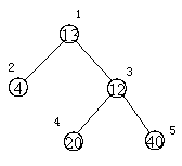# 【SSL 1614】医院设置【最短路 Floyed】

## Description

1处，则距离和=4+12+220+240=136
3处，则距离和=4*2+13+20+40=81## Sample Input

5
13 2 3
4 0 0
12 4 5
20 0 0
40 0 0


## Sample Output

81


## CODE：

#include<iostream>
#include<cstdio>
#include<cstring>
using namespace std;
int n,x,y,v,a,sum,ans;
int main()
{
cin>>n;
memset(a,0x7f/3,sizeof(a));
for(int i=1;i<=n;i++)
{
cin>>v[i]>>x>>y;
a[i][x]=1;
a[x][i]=1;  //邻接矩阵
a[i][y]=1;
a[y][i]=1;
a[i][i]=0x7fffffff;  //设无穷大
}
for (int k=1;k<=n;k++)
for (int i=1;i<=n;i++)  //floyed
for (int j=1;j<=n;j++)
a[i][j]=min(a[i][j],a[i][k]+a[k][j]);
ans=0x7fffffff;
for (int i=1;i<=n;++i)
{
sum=0;
for (int j=1;j<=n;++j)
sum+=v[j]*a[i][j];  //累加
ans=min(ans,sum);  //求最小
}
cout<<ans;
return 0;
}


©️2019 CSDN 皮肤主题: 黑客帝国 设计师: 上身试试